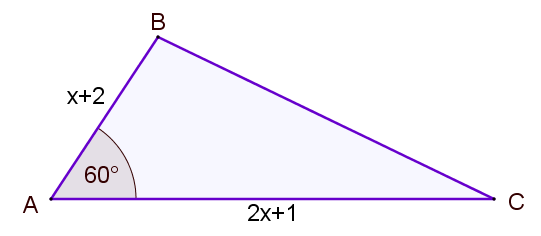# Exam-Style Questions.

## Problems adapted from questions set for previous Mathematics exams.

### 1.

GCSE Higher

(a) Write down the exact value of $$\tan 60^o$$.

(b) Find the exact area of this triangle.### 2.

GCSE HigherThe area of triangle ABC (not drawn to scale) is

$$\frac{35 \sqrt{3}}{4} m^2$$

If AB = $$x+2$$ metres and AC = $$2x+1$$ metres, find the value of $$x$$ to three significant figures.

The exam-style questions appearing on this site are based on those set in previous examinations (or sample assessment papers for future examinations) by the major examination boards. The wording, diagrams and figures used in these questions have been changed from the originals so that students can have fresh, relevant problem solving practice even if they have previously worked through the related exam paper.

The solutions to the questions on this website are only available to those who have a Transum Subscription.

Exam-Style Questions Main Page

Search for exam-style questions containing a particular word or phrase:

To search the entire Transum website use the search box in the grey area below.